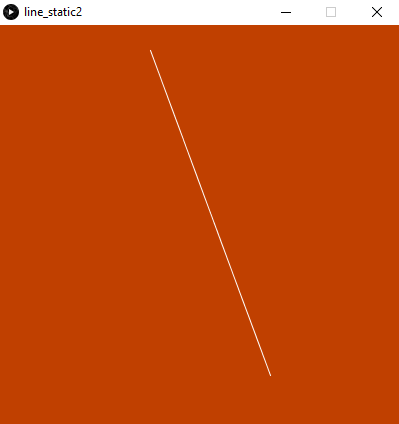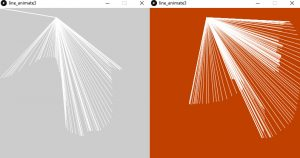# Processing.py: How to draw lines and use mouse events

Building on the first post about using Processing.py (shown in the table below), this post will demonstrate the basic structure of a Processing.py program, how to draw lines, and understand some other basics of the Processing.py programming language.

Processing.py: Getting Started

Example 1:

Drawing a line is as simple as providing two x, y points, one for each end of the line. So the syntax is `line(x1, y1, x2, y2)` . For example:

`line(15, 25, 70, 90)`

which produces:Example 2:

Create a static sketch that contains a line. In this example, we also set the size of the output window using `size()` , the color of the background using `background()`, and the color of the line using `stroke()`.

```size(400, 400)
background(192, 64, 0)
stroke(255)
line(150, 25, 270, 350)```

which produces:Example 3:

Use `setup()` and `draw()` functions to animate the line. Also use `mouseX` and `mouseY` events.

```def setup():
size(400, 400)
stroke(255)
background(192, 64, 0)

def draw():
line(150, 25, mouseX, mouseY)```

which produces:Example 4:

Animate a single line, where the top of the line is fixed but the bottom of the line moves with the mouse. This is done by moving the background from the setup function to the draw function. This change means that every time the mouse moves, the background is refreshed.

```def setup():
size(400, 400)
stroke(255)

def draw():
background(192, 64, 0)
line(150, 25, mouseX, mouseY)```

Example 5:

Create a new function, which is based on the `mousePressed()` event, where the background will change only after the mouse button is pressed. The result is that the sketch starts out with a grey background then turns orange when the mouse button is pressed. For example:

```def setup():
size(400, 400)
stroke(255)

def draw():
line(150, 25, mouseX, mouseY)

def mousePressed():
background(192, 64, 0)```

Outputs: